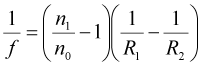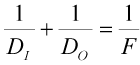# Lens Maker's Forumla#### n1 = index of refraction of a lens n0 = index of refraction of the medium surrounding the lens - usually air (n0=1) R1 = radius of curvature of the front surface R2 = radius of curvature of the second surface f = Focal Length

NOTE: The sign convention used is as follows: if R1 is positive, the first surface is convex, and if R1 is negative, the surface is concave. The signs are reversed for the back surface of the lens: if R2 is positive, the surface is concave, and if R2 is negative, the surface is convex.

 Index of refraction of the lens (n1): Radius of curvature (R1 in mm) Index of refraction of the surrounding medium (n0): Radius of Curvature (R2 in mm) Focal Length (in mm):

# Thin Lens Equation#### DI = Distance between the image and the center of the lens DO = Distance between the object and the center of the lens F = Focal length

NOTE: The sign convention used is as follows: if DI is negative then the image produced is a virtual image on the same side of the lens as the object itself.

 Object Distance (DO): Focal Length (F): Image Distance (DI): Magnification: